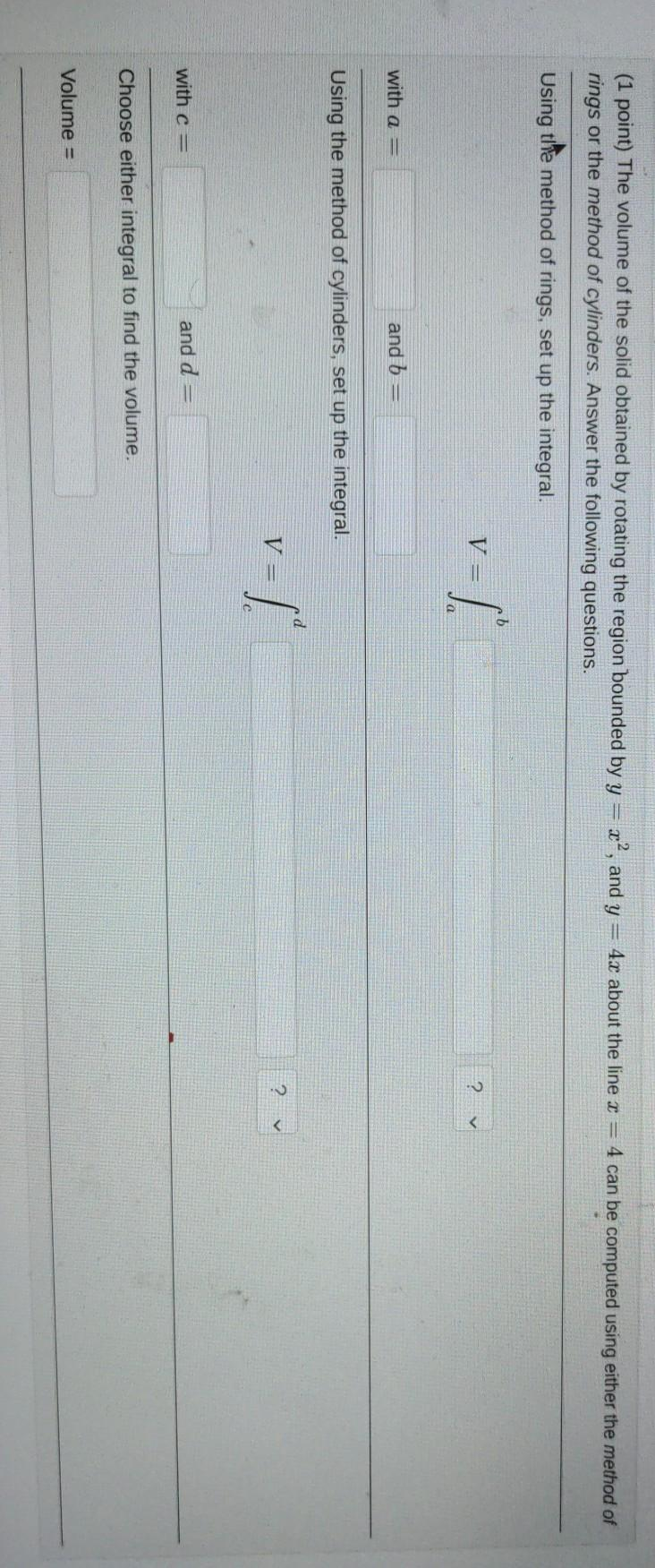Create an Account

Home / Questions / 4x about the line I = 4 can be computed using either the method of (1 point) The volume of...

4x about the line I = 4 can be computed using either the method of (1 point) The volume of the solid obtained by rotating the region bounded by y = x², and y rings or the method of cylinders. Answer t

4x about the line I = 4 can be computed using either the method of (1 point) The volume of the solid obtained by rotating the region bounded by y = x², and y rings or the method of cylinders. Answer the following questions. Using the method of rings, set up the integral. 5 1- with a = and b = Using the method of cylinders, set up the integral. d V = with c = and d = Choose either integral to find the volume. Volume =Apr 20 2021 View more View LessSubscribe To Get Solution